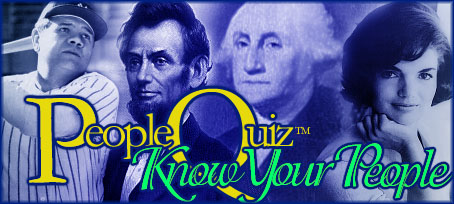.gsc-search-box { } Register for extra features!    LogonTrivia GamesBlogScoreboardsActivityCelebrity TriviaBiographiesPlayers
 Trivia Quiz - Mathematical Symbols How well do you know mathematical symbols? Quiz Number: 2006 Date Submitted: January 10, 2008 Quiz Categories: Science & Nature Quiz Type: General Quiz Author: BubblyJolie Average Score: 83.8 percent Times Taken: 162 times Taken by Registered Users: 20 Click here for the code snippet to embed this quiz in your website.(Image Source: Math Symbols)

Be sure to register and/or logon before taking quizzes to have your scores saved.

1. What does this mean? ∑
A.   Infinity
B.   equal to
C.   sum of
D.   parallel

2. What does this mean? ≠
A.   equal to
B.   not equal to
C.   defined as
D.   approaches

3. What does this mean? ≡
A.   plus or minus
B.   defined as
C.   approaches
D.   parallel

4. What does this mean? ≈
A.   equal to
B.   not equal to
C.   parallel
D.   aproximately equal to

5. What does this mean? ∞
A.   sum of
B.   absolute value
C.   less than
D.   infinity

6. What does this mean? ║
A.   perpendicular
B.   parallel
C.   less than
D.   infinity

7. What does this mean? ≥
A.   greater than or equal to
B.   infinity
C.   sum of
D.   equal to

8. What does this mean? ≤
A.   less than or equal to
B.   less than
C.   much less than
D.   approximately equal to

9. What does this mean? →
A.   approaches
B.   less than
C.   sum of
D.   plus or minus

10. What does this mean? ÷
A.   equal to
B.   not equal to
C.   defined as
D.   divide by